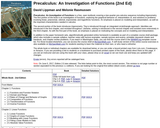Updating search results...

# 1 Result

View
Selected filters:
• Pierce CollegeConditional Remix & Share Permitted
CC BY-SA
Rating
0.0 stars

Precalculus: An Investigation of Functions is a free, open textbook covering a two-quarter pre-calculus sequence including trigonometry. The first portion of the book is an investigation of functions, exploring the graphical behavior of, interpretation of, and solutions to problems involving linear, polynomial, rational, exponential, and logarithmic functions. An emphasis is placed on modeling and interpretation, as well as the important characteristics needed in calculus.

Access also available here: http://www.opentextbookstore.com/precalc/

Chapter 1: Functions
Chapter 2: Linear Functions
Chapter 3: Polynomial and Rational Functions
Chapter 4: Exponential and Logarithmic Functions
Chapter 5: Trigonometric Functions of Angles
Chapter 6: Periodic Functions
Chapter 7: Trigonometric Equations and Identities
Chapter 8: Further Applications of Trigonometry

Subject:
Calculus
Mathematics
Material Type:
Textbook
Provider:
Open Textbook Network
Provider Set:
Pierce College
Author:
David Lippman
Melonie Rasmussen